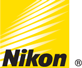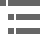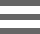## Numerical Aperture Light ConesThe light-gathering ability of a microscope objective is quantitatively expressed in terms of the numerical aperture, which is a measure of the number of highly diffracted image-forming light rays captured by the objective. Higher values of numerical aperture allow increasingly oblique rays to enter the objective front lens, producing a more highly resolved image.

This interactive tutorial demonstrates the change in numerical aperture light cones displayed by a microscope objective with corresponding changes in the angular aperture (and numerical aperture) of an objective. To operate this tutorial, use the mouse cursor to adjust the position of the Angular Aperture slider. As the angular aperture is varied with the slider, the size and shape of the illumination cone entering the objective front lens is altered. The adjustable numerical aperture range for this tutorial is from 0.13 to 0.95, and an approximate objective magnification factor has been assigned to each numerical aperture value.

The brightness of an image formed by an objective at a fixed magnification increases with the diameter of the angular aperture (the angle of the cone of light collected by the objective). Light rays emanating from the specimen proceed through air (or an immersion medium) that lies between the cover glass and the objective front lens. The angular aperture is expressed as the angle between the microscope optical axis and the direction of the most oblique light rays captured by the objective (see the tutorial figure). Mathematically, the numerical aperture is expressed as:

where n is the refractive index of the media in the object space (between the cover glass and the objective front lens) and θ is one-half the angular aperture. The value of n varies between 1.0 for air and 1.52 for a majority of immersion oils utilized in optical microscopy. The angular aperture, which varies with the objective focal length, is the maximum angle of image-forming light rays emanating from the specimen that the objective front lens can capture when the specimen is focused. As the objective focal length decreases, the maximum angle between the specimen and the outside diameter of the objective front lens increases, causing a proportionate increase in the angular aperture. From the equation above, it is obvious that numerical aperture increases with both angular aperture and the refractive index of the imaging medium.

Theoretically, the highest angular aperture obtainable with a standard microscope objective would be 180 degrees, resulting in a value of 90 degrees for the half-angle utilized in the numerical aperture equation. The sine of 90 degrees is one, which indicates that numerical aperture is limited not only by the angular aperture, but also by the imaging medium refractive index. A majority of microscope objectives are designed to operate with air (which has a refractive index of 1.0) as the imaging medium between the cover glass and the objective front lens. This yields a theoretical maximum numerical aperture of 1.00, but in actual practice, the highest numerical aperture for a dry objective is about 0.95 (the angular aperture half-angle equals approximately 72 degrees).

### Contributing Authors

Michael W. Davidson - National High Magnetic Field Laboratory, 1800 East Paul Dirac Dr., The Florida State University, Tallahassee, Florida, 32310.

# Microscopy U - The source for microscopy education•••